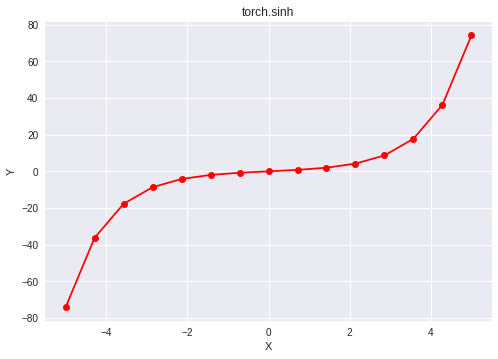# Python | PyTorch sinh() method

PyTorch is an open-source machine learning library developed by Facebook. It is used for deep neural network and natural language processing purposes.

The function `torch.sinh()` provides support for the hyperbolic sine function in PyTorch. It expects the input in radian form. The input type is tensor and if the input contains more than one element, element-wise hyperbolic sine is computed.

Syntax: torch.sinh(x, out=None)

Parameters:
x: Input tensor
name (optional): Output tensor

Return type: A tensor with the same type as that of x.

Code #1:

 `# Importing the PyTorch library ` `import` `torch ` ` `  `# A constant tensor of size 6 ` `a ``=` `torch.FloatTensor([``1.0``, ``-``0.5``, ``3.4``, ``-``2.1``, ``0.0``, ``-``6.5``]) ` `print``(a) ` ` `  `# Applying the sinh function and ` `# storing the result in 'b' ` `b ``=` `torch.sinh(a) ` `print``(b) `

Output:

``` 1.0000
-0.5000
3.4000
-2.1000
0.0000
-6.5000
[torch.FloatTensor of size 6]

1.1752
-0.5211
14.9654
-4.0219
0.0000
-332.5700
[torch.FloatTensor of size 6]
```

Code #2: Visualization

 `# Importing the PyTorch library ` `import` `torch ` ` `  `# Importing the NumPy library ` `import` `numpy as np ` ` `  `# Importing the matplotlib.pylot function ` `import` `matplotlib.pyplot as plt ` ` `  `# A vector of size 15 with values from -5 to 5 ` `a ``=` `np.linspace(``-``5``, ``5``, ``15``) ` ` `  `# Applying the hyperbolic sine function and ` `# storing the result in 'b' ` `b ``=` `torch.sinh(torch.FloatTensor(a)) ` ` `  `print``(b) ` ` `  `# Plotting ` `plt.plot(a, b.numpy(), color ``=` `'red'``, marker ``=` `"o"``)  ` `plt.title(``"torch.sinh"``)  ` `plt.xlabel(``"X"``)  ` `plt.ylabel(``"Y"``)  ` ` `  `plt.show() `

Output:

```-74.2032
-36.3203
-17.7696
-8.6771
-4.2032
-1.9665
-0.7766
0.0000
0.7766
1.9665
4.2032
8.6771
17.7696
36.3203
74.2032
[torch.FloatTensor of size 15]
```My Personal Notes arrow_drop_upCheck out this Author's contributed articles.

If you like GeeksforGeeks and would like to contribute, you can also write an article using contribute.geeksforgeeks.org or mail your article to contribute@geeksforgeeks.org. See your article appearing on the GeeksforGeeks main page and help other Geeks.

Please Improve this article if you find anything incorrect by clicking on the "Improve Article" button below.

Article Tags :

Be the First to upvote.

Please write to us at contribute@geeksforgeeks.org to report any issue with the above content.